Question

A simple random sample of 22 full IQ scores for the group with medium exposure to lead was taken. The sample standard deviation of this sample is 14.3. Construct a 95% confidence interval estimate for the population standard deviation.

Requirements:

a) Simple random sample

b) Check normality

given data are:-

sample size (n ) = 22

sample sd (s) = 14.3

the chi square critical value table be:-

 critical value excel function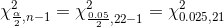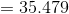=CHISQ.INV.RT(0.025,21)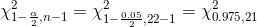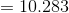=CHISQ.INV.RT(0.975,21)

the 95% confidence interval for the population standard deviation be:-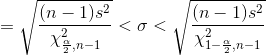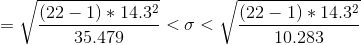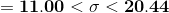*** if you have any doubt regarding the problem please write it in the comment box.if you are satisfied please give me a LIKE if possible...

#### Earn Coins

Coins can be redeemed for fabulous gifts.

Similar Homework Help Questions
• ### Confidence Interval about a Population Standard Deviation 3) Consider the same information as in Example 2...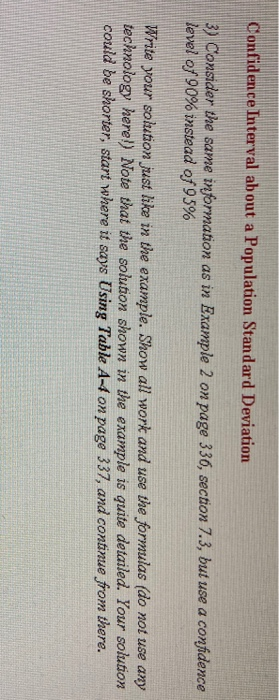Confidence Interval about a Population Standard Deviation 3) Consider the same information as in Example 2 on page 336, section 7.3, but use a confidence level of 90% instead of 95% Write your solution just like in the example. Show all work and use the formulas (do not use any technology here!) Note that the solution shown in the example is quite detailed. Your solution could be shorter, start where it says Using Table A-4 on page 337, and continue...

• ### Listed in the data table are 10 scores for a random sample of subjects with medium...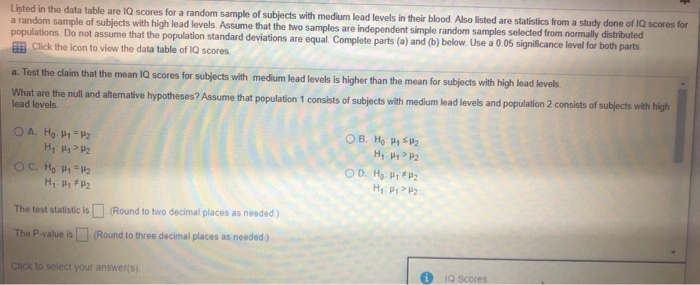Listed in the data table are 10 scores for a random sample of subjects with medium load levels in their blood. Also listed are statistics from a study done of IQ scores for a random sample of subjects with high lead levels. Assume that the two samples are independent simple random samples selected from normally distributed populations. Do not assume that the population standard deviations are equal. Complete parts (a) and (b) below. Use a 0.05 significance level for both...

• ### The IQ scores for a random sample of subjects with mediu lead levels in their blood and another r...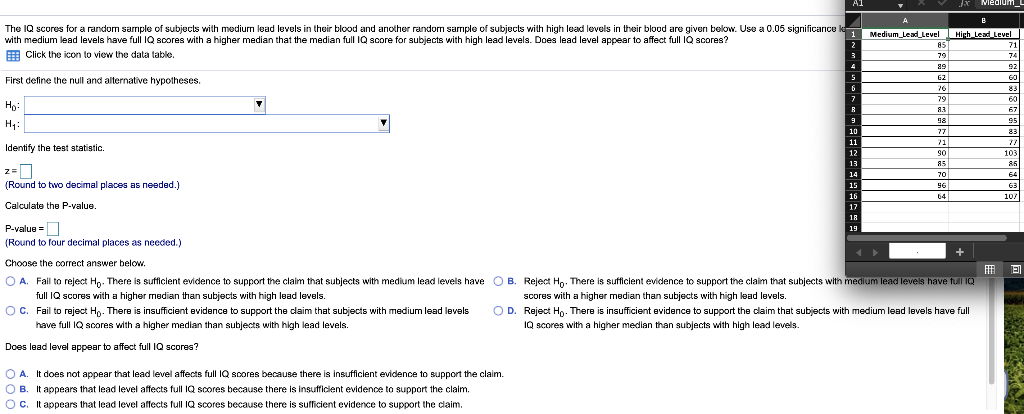18 The IQ scores for a random sample of subjects with mediu lead levels in their blood and another random sample of subjects with high lead levels in their blood are given below. Use a 0.05 significance Medium Lead Level Hich with medium lead levels have full Q scores with a higher median that the median full IQ score for subjects with high lead levels. Does lead level appear to affect full IQ scores? EEB Click the icon to view...

• ### Listed in the data table are IQ scores for a random sample of subjects with medium...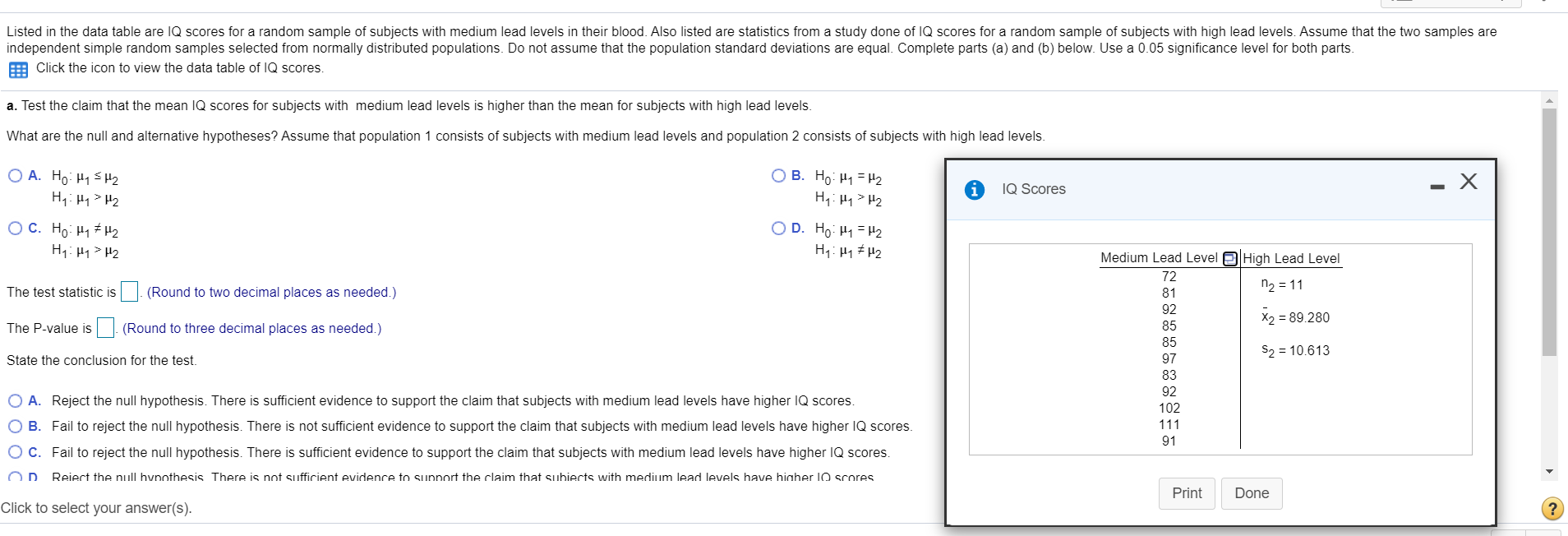Listed in the data table are IQ scores for a random sample of subjects with medium lead levels in their blood. Also listed are statistics from a study done of IQ scores for a random sample of subjects with high lead levels. Assume that the two samples are independent simple random samples selected from normally distributed populations. Do not assume that the population standard deviations are equal. Complete parts (a) and (b) below. Use a 0.05 significance level for both...

• ### 10 11 A psychologist wants to estimate the standard deviation of IQ scores. It is widely...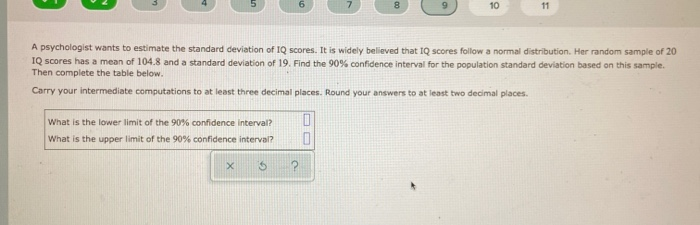10 11 A psychologist wants to estimate the standard deviation of IQ scores. It is widely believed that IQ scores follow a normal distribution. Her random sample of 20 IQ scores has a mean of 104.8 and a standard deviation of 19. Find the 90% confidence interval for the population standard deviation based on this sample. Then complete the table below. Carry your intermediate computations to at least three decimal places. Round your answers to at least two decimal places....

• ### A simple random sample of size n is drawn from a population that is normally distributed....

A simple random sample of size n is drawn from a population that is normally distributed. The sample mean, x̅, is found to be 107 , and the sample standard deviation, s, is found to be 10 .(a) Construct a 98 % confidence interval about μ if the sample size, n, is 22 .(b) Construct a 98 % confidence interval about μ if the sample size, n, is 12 .(c) Construct a 95 % confidence interval about μ if the...

• ### Medium lead level: 72,98,92,85,82,97,83,92,102,111,91 & high lead level: n2=11 , x2=90.267 , s2=10.588 %) 9.2.12-T EQuestion...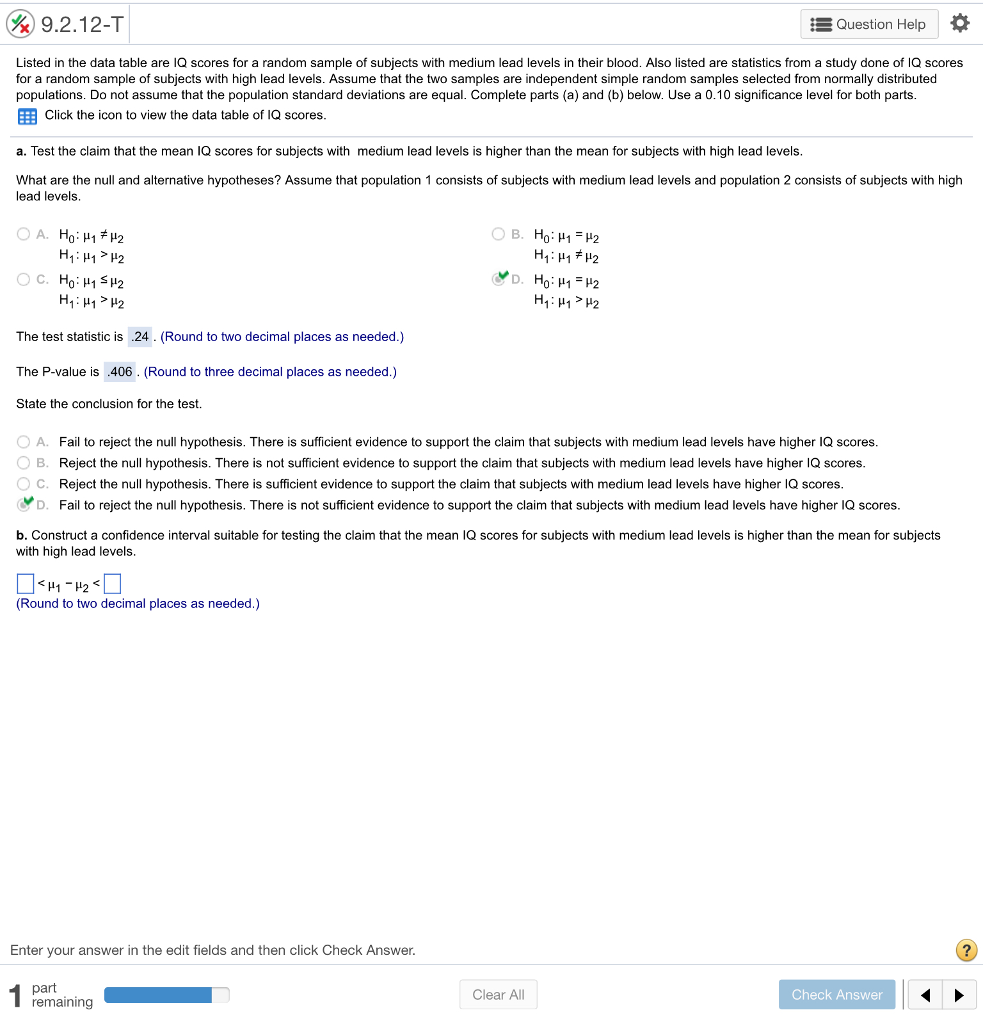Medium lead level: 72,98,92,85,82,97,83,92,102,111,91 & high lead level: n2=11 , x2=90.267 , s2=10.588 %) 9.2.12-T EQuestion Help Listed in the data table are IQ scores for a random sample of subjects with medium lead levels in their blood. Also listed are statistics from a study done of IQ scores for a random sample of subjects with high lead levels. Assume that the two samples are independent simple random samples selected from normally distributed populations. Do not assume that the population...

• ### 4) Assume that IQ scores of college graduates are normally distributed. A researcher collects a random...

4) Assume that IQ scores of college graduates are normally distributed. A researcher collects a random sample of 25 college graduates and tests their IQ. The sample had a mean IQ of 108 with a standard deviation of 15.6. a) Find a 95% confidence interval for the true mean IQ for college graduates. b) Provide the margin of error of the interval as your answer. Round your answer to 1 decimal place.

• ### 26. Birth Weights A simple random sample of birth weights in the United States has a mean of 3433...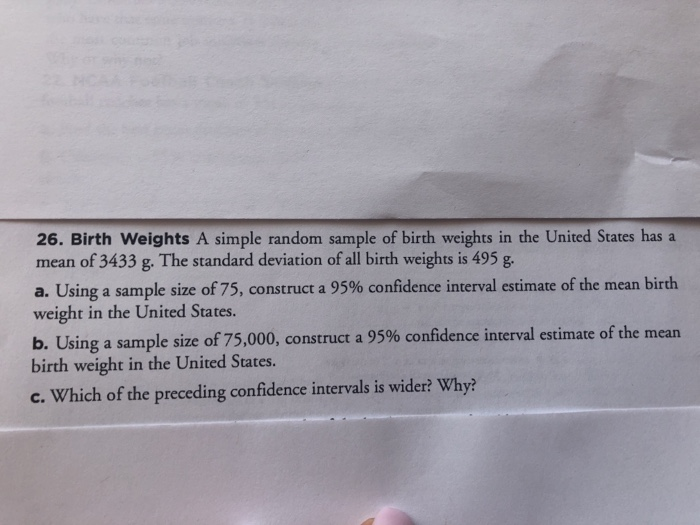*without using interval functions on calculator* 26. Birth Weights A simple random sample of birth weights in the United States has a mean of 3433 g. The standard deviation of all birth weights is 495 g a. Using a sample size of 75, construct a 95% confidence interval estimate of the mean birth weight in the United States. b. Using a sample size of75,000, construct a 95% confidence interval estimate of the mean birth weight in the United States. c....

• ### A simple random sample from a population with a normal distribution of 108 body temperatures has...

A simple random sample from a population with a normal distribution of 108 body temperatures has x =98.40 °F and s =0.67°F. Construct a 95% confidence interval estimate of the standard deviation of body temperature of all healthy humans. Is it safe to conclude that the population standard deviation is less than 1.10°F